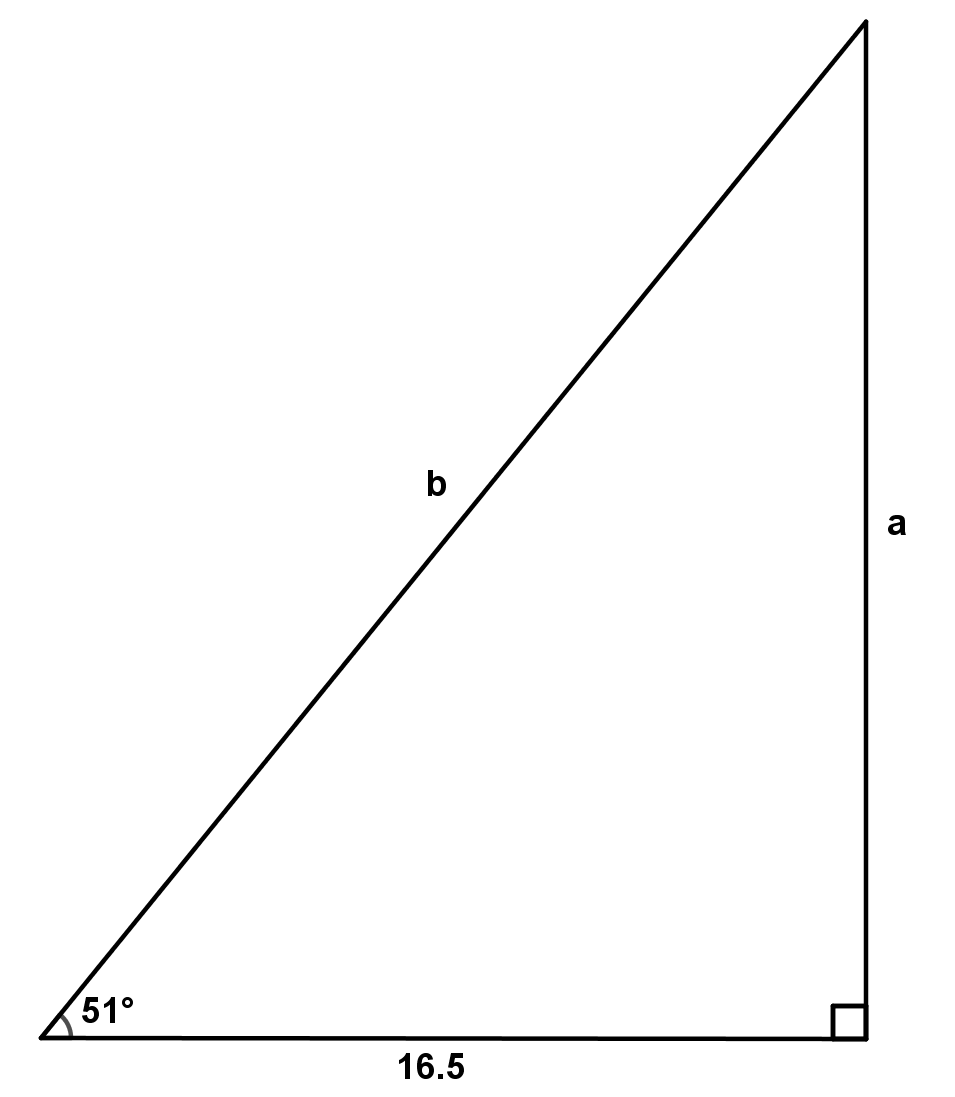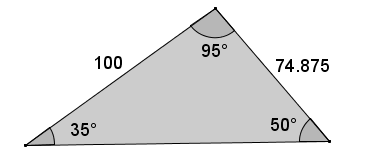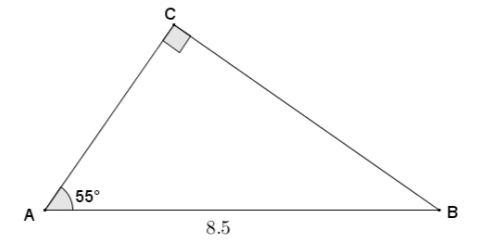# Right Triangles and Trigonometry

## Objective

Solve for missing sides of a right triangle given the length of one side and measure of one angle.

## Common Core Standards

### Core Standards

?

• G.SRT.C.8 — Use trigonometric ratios and the Pythagorean Theorem to solve right triangles in applied problems. Modeling is best interpreted not as a collection of isolated topics but in relation to other standards. Making mathematical models is a Standard for Mathematical Practice, and specific modeling standards appear throughout the high school standards indicated by a star symbol (★). The star symbol sometimes appears on the heading for a group of standards; in that case, it should be understood to apply to all standards in that group.

?

• 8.G.B.7

## Criteria for Success

?

1. Given any one side and any one angle measure, determine the rest of the side lengths and missing angle measures of a right triangle.
2. Solve triangles using the Pythagorean theorem, similarity relationships, and trigonometric ratios.
3. Understand that trigonometric ratios and the Pythagorean theorem can only be used to solve RIGHT triangles.

## Tips for Teachers

?

• In later lessons, students in advanced courses will learn the Law of Sines and the Law of Cosines.  Criteria for Success #3 is necessary to include due to students appropriately using the trigonometric ratios in non-right triangles before they know about the Law of Sines and Law of Cosines.
• Students should use their calculators with trigonometric ratios in this lesson. Ensure that students are familiar with how to use their calculators to calculate the trigonometric ratios.

## Anchor Problems

?

### Problem 1

Below is a triangle with angle measure 51° and side length of measure 16.5.  Find the missing side lengths and angle measures.### Problem 2

A shipmate set a boat to sail exactly 27° NE from the dock.  After traveling 120 miles, the shipmate realized he had misunderstood the instructions from the captain; he was supposed to set sail going directly east! The boat is now due north of his destination.

a) How many miles would the boat have traveled if the shipmate followed the directions of the captain?
b) How many miles is the boat off course after traveling for 120 miles at 27° NE?

#### References

EngageNY Mathematics Geometry > Module 2 > Topic E > Lesson 28Exercise 5

Geometry > Module 2 > Topic E > Lesson 28 of the New York State Common Core Mathematics Curriculum from EngageNY and Great Minds. © 2015 Great Minds. Licensed by EngageNY of the New York State Education Department under the CC BY-NC-SA 3.0 US license. Accessed Dec. 2, 2016, 5:15 p.m..

Modified by The Match Foundation, Inc.

### Problem 3

Find the base of the triangle:#### References

EngageNY Mathematics Geometry > Module 2 > Topic E > Lesson 28Example 2 - Image

Geometry > Module 2 > Topic E > Lesson 28 of the New York State Common Core Mathematics Curriculum from EngageNY and Great Minds. © 2015 Great Minds. Licensed by EngageNY of the New York State Education Department under the CC BY-NC-SA 3.0 US license. Accessed Dec. 2, 2016, 5:15 p.m..

## Problem Set

?

The following resources include problems and activities aligned to the objective of the lesson that can be used to create your own problem set.

• Include problems with non-right triangles as error analysis problems. Ask students to identify why they are unable to use the trigonometric ratios to find the values of the missing sides in non-right triangles.
• Include problems where right triangles are embedded in other right triangles and students need to investigate the diagram carefully to find the solution.
• Include problems where students use a trigonometric ratio of one triangle to find the missing side length of a similar triangle.

Given right triangle ${A{BC}}$ with hypotenuse ${AB=8.5}$ and ${m\angle A=55^\circ}$, find ${AC}$ and ${BC}$ to the nearest hundredth.